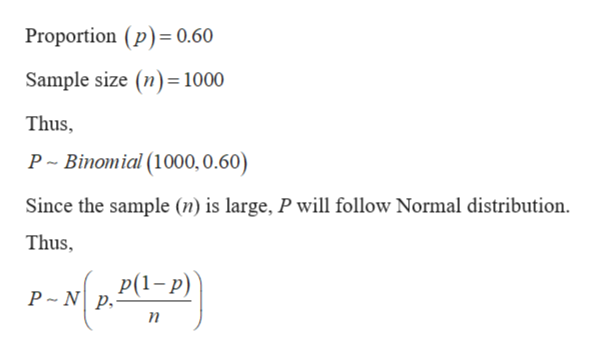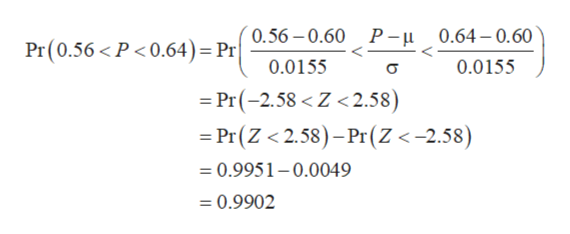# suppose that 60% of all students currently enrolled at all California Community College campuses combined are female. Researchers want to do a survery, and randomly select 1000 students who are currently enrolled at CSU campuses. Let P represent the proportion of students in the sample who are female. d. find the probability that more than 65% in the sample are female. Use the distribution Pe. find the probability that between 56% and 64% in the sample are female. Use the distribution Pf. repreat part (e), but use the Normal approximation to the binomial without  making the continuity correction. Show your calculator input and calculations or reasoning

Question

suppose that 60% of all students currently enrolled at all California Community College campuses combined are female. Researchers want to do a survery, and randomly select 1000 students who are currently enrolled at CSU campuses. Let P represent the proportion of students in the sample who are female.

d. find the probability that more than 65% in the sample are female. Use the distribution P

e. find the probability that between 56% and 64% in the sample are female. Use the distribution P

f. repreat part (e), but use the Normal approximation to the binomial without  making the continuity correction. Show your calculator input and calculations or reasoning

check_circle

Step 1

It is provided that P is defined as the proportion of students in the sample who are female. The proportion of females, who are enrolled in California Community college campuses, is 60%.help_outlineImage TranscriptioncloseProportion (p) 0.60 Sample size (n) 1000 Thus, P Binomial (1000, 0.60) Since the sample (n) is large, P will follow Normal distribution Thus Р(1-р) P N p п fullscreen
Step 2

d)

The probability, that more than 65.00% of the students in the sample are females, can be calculated as:

Step 3

e)

The probability, that more than 56.00% and less than 64.00% of the...help_outlineImage TranscriptionclosePr(0.56 P<0.64)= Pr0.56-0.60 P- 0.64-0.60 0.0155 0.0155 Pr(-2.58 < Z< 2.58) = Pr(Z < 2.58)-Pr(Z <-2.58) =0.9951-0.0049 =0.9902 fullscreen

### Want to see the full answer?

See Solution

#### Want to see this answer and more?

Solutions are written by subject experts who are available 24/7. Questions are typically answered within 1 hour.*

See Solution
*Response times may vary by subject and question.
Tagged in

### Other